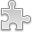# Courses / Module

Toggle Print

##FINITE MATHEMATICS

Module code: MT241P
Credits: 5
Semester: 1
Department: MATHEMATICS AND STATISTICS
International:Overview

Module Objective:
The aim of this module is to further to students knowledge of Finite Mathematics.

Number theory: Induction. Division algorithm, g.c.d., Euclidean algorithm, linear Diophantine equations, primes, Euclid''s lemma, Fundamental Theorem of Arithmetic, Modular arithmetic, congruences, solving linear congruences, divisibility tests, Chinese remainder theorem. Complex numbers: multiplication and De Moivre''s theorem. Set theory: product, relations, equivalence relations. Combinatorics and Probability: Applications of probability. Terminology: experiment, sample space, event. Assigning probabilities: equally likely outcomes, long run frequency. Axioms of probability. Consequences of axioms. Examples. Equiprobable outcomes: Random sampling without replacement. Equiprobable outcomes: Random sampling with replacement. The birthday problem. Conditional probability, independence. Law of total probability. Bayes theorem and examples. Discrete random variables. Probability mass function, probability histogram. Expected values, mean, variance and standard deviation. Bernoulli trials, Binomial distribution. Mean and variance of the binomial distribution. The Poisson distribution.Learning OutcomesTeaching & Learning methodsAssessmentAutumn Supplementals/ResitsPre-RequisitesTimetable